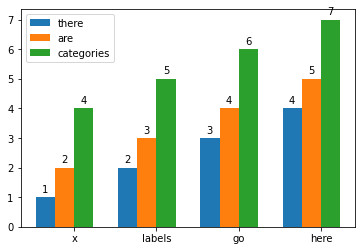# Grouped Bar Plot

Published:

I often have a need to plot a grouped bar plot. Matplotlib provides this example, which is helpful, but not quite generalizable enough for my needs, as it only shows how to group 2 categories together. Here is a generalization of that tutorial that was very helpful for me and I hope is helpful for others as well.

import matplotlib.pyplot as plt
import numpy as np

from typing import List, Optional

def grouped_barplot(
data,
clabels: List[str],
xlabels: List[str],
gap: float = 0.3,
show_legend: bool = True,
show_bar_labels: bool = True,
ax: Optional[plt.Axes] = None,
):
"""

Parameters
----------
data: array-like
size=(len(clabels), len(xlabels))
clabels list(str):
xlabels: list(str)
gap: float
Gap between categories
show_legend: bool
Show legend. Default = True
show_bar_labels: bool
Show data values above each bar. Default = True
ax: plt.Axes
If not provided, a new figure will be created.

Returns
-------
ax, all_rects

"""

if ax is None:
_, ax = plt.subplots()

x = np.arange(len(xlabels))  # the label locations
width = (1 - gap) / len(clabels)  # the width of the bars

all_rects = []
for i, (cdata, clabel) in enumerate(zip(data, clabels)):
rects = ax.bar(x - .5 + gap / 2 + i * width, cdata, width, label=clabel)
if show_bar_labels:
all_rects.append(rects)

# Add some text for labels, title and custom x-axis tick labels, etc.
ax.set_xticks(x, xlabels)
if show_legend:
ax.legend()

return ax, all_rects


Example usage:

grouped_barplot(
data=[[1,2,3,4], [2,3,4,5], [4,5,6,7]],
clabels=["there", "are", "categories"],
xlabels=["x", "labels", "go", "here"],
)Tags: